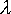QUESTION #406

# Is there any gravitational force between two photons?

Now photons, though technically massless, do carry energy. The energy of a photon with a wavelength of 560 nanometers ('yellow light') is given by E=hc/where E is the energy, h is planck's constant (6.626x10-34 J*s), c is the speed of light, andis the wavelength of the light. The energy of our photon is thus 3.55x10-19 J. Note that this is 35 or so orders of magnitude below mass energy.